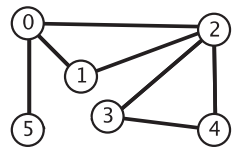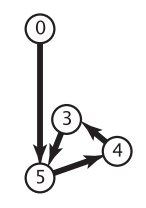# How to implement detection of undirected and directed loops in Java

## Undirected rings

An undirected graph with rings is shown below, where there are two rings, 0-2-1-0 and 2-3-4-2, respectively.To detect rings in an undirected graph, you can use depth-first search. Suppose we start from vertex 0, then walk to the adjacent vertex 2, then walk to the vertex 1 adjacent to vertex 2. Since vertex 0 and vertex 1 are adjacent and vertex 0 is labeled, it means we have spared a loop, so there is a loop in the undirected graph. Although vertex 2 is adjacent to vertex 1, it does not indicate the existence of a ring, because we go directly from vertex 2 to vertex 1, which is only an edge relationship. The algorithm is shown below.

``````package com.javaisland.graph;

import com.javaisland.collection.stack;

/**
* The ring in the undirected graph
*/
public class Cycle {
private boolean[] marked;
private Graph graph;
private boolean hasCycle;

public Cycle(Graph graph) {
this.graph = graph;
marked = new boolean[graph.V()];

for (int v = 0; v < graph.V(); ++v) {
if (!marked[v]) {
dfs(v);
}
}
}

private void dfs(int s) {
if (hasCycle()) return;

vertexes.push(s);
marked[s] = true;

int lastVertex = s;
while (!vertexes.isEmpty()) {
int v = vertexes.pop();

for (int w : graph.adj(v)) {
if (!marked[w]) {
marked[w] = true;
vertexes.push(w);
} else if (w ! = lastVertex) {
hasCycle = true;
return;
}
}

lastVertex = v;
}
}

/**
* Whether there is a ring in the graph
*/
public boolean hasCycle() {
return hasCycle;
}
}
``````

## Directed rings

### Directed graphs

The implementation of a directed graph is almost the same as the implementation of an undirected graph in the previous blog “How to Implement Undirected Graphs in Java”, except that when adding edges v-w, only vertex w is added to the chain of vertices v, and the chain of vertices w is not without manipulating the chain table of vertex w. If you change the access rights of the member variables in `LinkGraph` to `protected`, you can simply inherit and override the `addEdge` method.

``````package com.javaisland.graph;

super(V);
}

@Override
public void addEdge(int v, int w) {
E++;
}

@Override
public Digraph reverse() {
for (int v = 0; v < V(); ++v) {
for (int w : adj(v)) {
}
}
return digraph;
}
}
``````

### Detection algorithm

A directed graph containing a directed ring is shown below, where 5-4-3-5 form a ring of.A recursive implementation of depth-first search is used here to detect directed rings. Suppose we start at vertex 0, go through vertices 5, 4, and 3, and eventually come across vertex 5, which is adjacent to vertex 3. At this point, if we know that vertex 5 has been visited and the recursive function is still pressed on the stack, it means that the depth-first search starts at vertex 5 and goes back to vertex 5, i.e., it finds the directed ring. The algorithm is shown below.

``````package com.javaisland.graph;

import com.javaisland.collection.stack;

/**
* The ring in the directed graph
*/
public class DirectedCycle {
private boolean[] marked;
private boolean[] onStack;
private int[] edgeTo;
private Graph graph;
private Stack<Integer> cycle;

public DirectedCycle(Digraph graph) {
this.graph = graph;
marked = new boolean[graph.V()];
onStack = new boolean[graph.V()];
edgeTo = new int[graph.V()];

for (int v = 0; v < graph.V(); ++v) {
if (!marked[v]) {
dfs(v);
}
}
}

private void dfs(int v) {
marked[v] = true;
onStack[v] = true;

for (int w : graph.adj(v)) {
if (hasCycle()) return;
if (!marked[w]) {
marked[w] = true;
edgeTo[w] = v;
dfs(w);
} else if (onStack[w]) {
cycle.push(w);
for (int i = v; i ! = w; i = edgeTo[i]) {
cycle.push(i);
}
cycle.push(w);
}
}

onStack[v] = false;
}

/**
* Whether there is a ring in the graph
*/
public boolean hasCycle() {
return cycle ! = null;
}

/**
* A loop in the graph
*/
public Iterable<Integer> cycle() {
return cycle;
}
}
``````